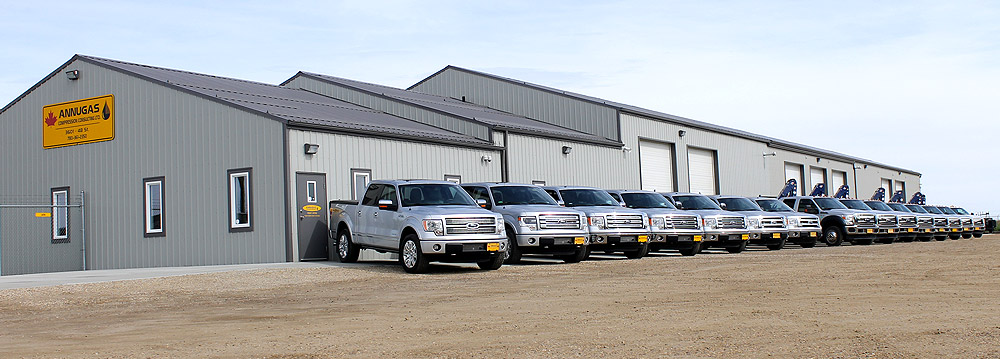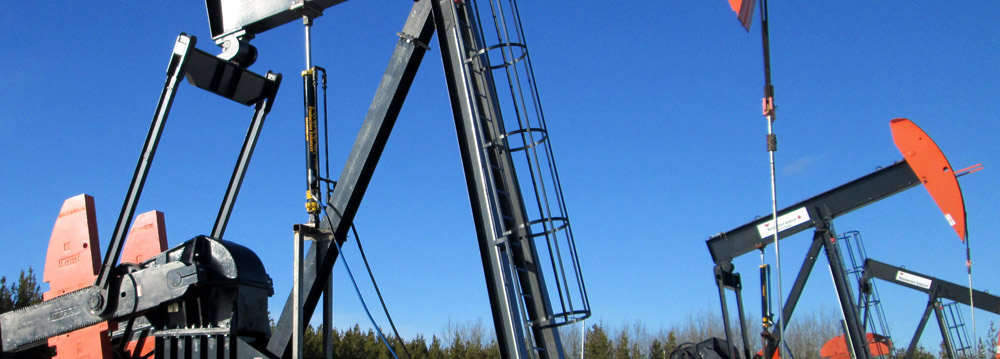# 1.866.266.8427# TECHNICAL INFO

## Sizing Formula

By using the formula below, you can arrive at the pressure (PSIA) that an Annugas Production Enhancer® will draw the casing pressure down to.

 Formula: MCF/D x 1600 = PSIA S X L X A

MCF/D is the gas production of the well.

 Where S = Strokes/minute of walking beam L = Length of stroke of Annugas in feet A = Area of Annugas piston in Compression Area (in2) P = Suction pressure absolute (PSIA) And: Diam. of Piston (in.) Compression Area (in2) 5 34.4 6 51.5 7 72.1 8 95.5 10 152.0

 Example (Model AG648) S=6, L=4, A=51.5 12MCF/D 12 X 1600 = 15.5 PSIA 6 x 4 x 51.5

Subtract absolute pressure (14) to get gauge reading.